# Multiplication and Division by 10, 100 etc & Adding and Subtracting Decimals

## Multiplication and Division by 10, 100 etc

We are all able to multiply and divide by powers of ten easier than any other numbers as the digits being dealt with remain in the same order. Children often struggle to come to terms with this and still work out '1 x ...' or try to find the position of each digit when they could just write the digits out from start to finish in one go.

When we were taught the normal way tended to be to refer to moving the decimal point. For various reasons this has changed in recent years and we now refer to keeping the decimal point in the same place but moving the numbers.

To multiply by 10, 100, 1000 etc we move the digits to the left by however many zeroes there are in the number we are multiplying by. This will automatically make the number larger.

For example: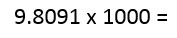The digits stay in the same order and, with the decimal point staying where it is, they all move three places to the right. It will be three places because 1000 has three zeroes.

The answer, therefore, is 9809.1

With division, the same rules apply but instead of moving to the left, we move the digits to the right. This will automatically make the number smaller.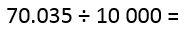Keep the numbers in the same order and that includes any zeroes. 10 000 has four zeroes so we move the digits four places to the right.

The answer, therefore, is 0.0070035

The easiest technique is to find the position of the first digit and simply write the remaining ones after it.

## Adding and Subtracting Decimals

The concept is simple when it's all about adding money, but get children to add and subtract decimals out of context and they can't see how easy it is!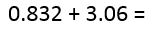The column addition method is straightforward enough and applies to decimals as well as whole numbers. The danger for the child is that they will ignore the decimal points or not line them up accurately. If the decimal point is written in when the first number is written on the page, immediately write in the second decimal point exactly underneath the first.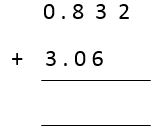At this point you may want to put a zero under the two, just to avoid confusion. With addition, though, it isn't essential. After this, simply carry out a normal addition sum and insert the decimal point under the others.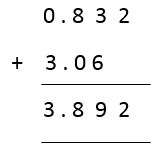I always encourage children to estimate the answer when dealing with decimals and place value - get them to imagine what the approximate answer will be. In this case, they should think 'just under 4' as an appropriate guess and the answer confirms this. If they get an answer which is way off the mark, they must be prepared to redo the sum.

Subtraction works in the same way.In this case we should really put a zero in under the two to make things more concrete. We can then move along the line of digits, subtracting each as we go. 2 - 0 = 2; 9 - 6 = 3; 8 - 1 = 7; 4 - 0 = 4.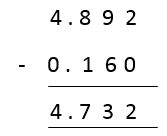Again, estimate in advance to avoid any wildly wrong answers sneaking through.

We use cookies to make your experience of our website better.

To comply with the new e-Privacy directive, we need to ask for your consent -